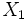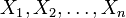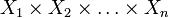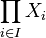# Acyclicity is product-closed

This article gives the statement, and possibly proof, of a topological space property (i.e., acyclic space) satisfying a topological space metaproperty (i.e., product-closed property of topological spaces)
View all topological space metaproperty satisfactions | View all topological space metaproperty dissatisfactions
Get more facts about acyclic space |Get facts that use property satisfaction of acyclic space | Get facts that use property satisfaction of acyclic space|Get more facts about product-closed property of topological spaces

## Statement

### For two spaces

Suppose$X_1$ and$X_2$ are topological spaces that are both acyclic spaces. Then, the product space$X_1 \times X_2$, endowed with the product topology, is also an Acyclic space (?).

### For finitely many spaces

Suppose$X_1, X_2, \dots, X_n$ are topological spaces that are all acyclic spaces. Then, the product space$X_1 \times X_2 \times \dots \times X_n$, endowed with the product topology, is also an acyclic space.

### For an arbitrary number of spaces

Suppose$X_i, i \in I$, are topological spaces that are all acyclic spaces. Then, the product space$\prod_{i \in I} X_i$, endowed with the product topology, is also an acyclic space.